Join Us | Latest Articles | ContactJournal Home Editorial Board Archive Submit to this journal Current issue

## Noise Analysis of Gene Regulatory Networks Using Particle Filter

### Haixin Wang* and Dawit Aberra

Department of Mathematics and Computer Science, Fort Valley State University, USA

*Corresponding author: Haixin Wang, Department of Mathematics and Computer Science, Fort Valley State University, Fort Valley, GA 31030, USA, Tel: 478-827-3149, Fax: 478-825-6286, E-mail: wangh@fvsu.edu
Int J Clin Biostat Biom, IJCBB-1-006, (Volume 1, Issue 1), Original Article; ISSN: 2469-5831
Received: August 10, 2015 | Accepted: October 01, 2015 | Published: October 03, 2015
Citation: Wang H, Aberra D (2015) Noise Analysis of Gene Regulatory Networks Using Particle Filter. Int J Clin Biostat Biom 1:006. 10.23937/2469-5831/1510006
Copyright: © 2015 Wang H, et al. This is an open-access article distributed under the terms of the Creative Commons Attribution License, which permits unrestricted use, distribution, and reproduction in any medium, provided the original author and source are credited.

Abstract

One of the most important properties in gene expression is the stochasticity. Gene expression process is noisy and fluctuant. In this paper, the noise of gene regulatory networks (GRNs) using polynomial model and S-system model is analyzed by proposed approach on the basis of particle filter. The measurement noise and process noise are analyzed to test noise effects on the synthetic GRNs. The relation among Root Mean Square (RMS) error, measurement noise, and system noise covariance is analyzed. Microarray data are used to testify the noise analysis model by particle filter. We conclude that measurement noise is the main reason to incur the RMS error, which is in agreement with biological experimental results.

Keywords

Noise analysis, Particle filter, Polynomial, S-system, Gene regulatory networks

Introduction

One of the most important prosperities in gene expression is the stochasticity. Gene expression process is noisy and fluctuant.

The noise sources

Generally, noise sources can be partitioned into two categories [1-4]. Firstly, gene expression is a sequence of biochemical reactions which have inherent stochasticity. Those biochemical reactions depend on the molecular events and the difference in the internal states of cells like random births, deaths, and collisions of molecules . The inherent stochasticity in the system is named as intrinsic noise. Secondly, variabilities in factors external to the system also contribute the noise. The environment is complicated and the subtle environment difference may result in fluctuations in gene expression. Those kinds of noise sources are referred to as extrinsic noise. Generally, the noise effects of gene expression are the joint effects of intrinsic noise and extrinsic noise.

The concept of intrinsic noise and extrinsic noise has been proved experimentally [3,5]. Although experimentally, it is difficult to distinguish intrinsic noise from extrinsic noise in vivo . Experiments have shown that both intrinsic and extrinsic noise contribute substantially to the overall variation. Rapid fluctuations in mRNA are the sources of intrinsic noise . The experiments showed that noise in gene expression level caused the fluctuation in protein level in a clonal population of E. coli . Extrinsic noise is the primary source of stochastic fluctuation in gene expression, which is also observed in budding yeast .

Intrinsic noise: Internal noise is stochastic and inherent in the biochemical reactions such as transcription and translation. Its magnitude is proportional to the inverse of the system size and its origin is often thermal . The intrinsic noise is the stochastic events during the process of gene expression from level of promoter binding to mRNA translation to protein degradation. In single gene expression, the intrinsic noise comes from fluctuations generated by stochastic promoter activation, promoter inactivation, mRNA and protein production and delay .

Extrinsic noise: Extrinsic noise is the differences between cells either in local environment or in the concentration or activity of any factor that affects gene expression. The extrinsic noise is consequence of the fluctuations in the amounts or states of other cellular components such as molecular species and RNA polymerase. It originates in the random variation of one or more of the externally set control parameters [7,8]. Those kinds of noise vary from time to time, from cell to cell and gene sequence such as the number of RANPs or ribosomes, the stage in the cell cycle, the quantity of the protein, mRNA degration machinery and the cell environment, signals abundance of polymerase, ribosomes growth and division of the cell . Extrinsic noise can be divided into two categories : global noise, or fluctuations in the rates of the basic reactions that affect expression of all genes, and gene or pathway-specific extrinsic noise, such as fluctuations in the abundance of a particular transcription factor or stochastic events in a specific signal transduction pathway.

Noise effect

Noise or variation in the process of gene expression may contribute to the cells and organisms' variability . Both the magnitude and the frequency of the noise affect the consequence. Small changes in protein abundance may have dramatic effects on fitness if they persist long enough, whereas large fluctuations in abundance may not have any effect if they occur too frequently to affect a cellular process. The observation that the time scale for intrinsic noise fluctuations is much shorter than that for extrinsic noise suggests that extrinsic noise may affect cellular phenotype more strongly than intrinsic noise at least in E. coli . The presence of stochasticity in gene expression has been confirmed to result in noise in protein abundance, but other sources of noise may result in phenotype variability. Because cellular components interact with one another in complex regulatory networks, the fluctuation in amount of even a single component may affect the performance of the whole system. The mechanisms through which a natural genetic network can operate reliably despite noisy environments and stochasticity in gene expression are not known and remain a difficult challenge ion genetic network engineering .

Modeling of Gene Regulatory Networks with Noise

Gene expression data raise the possibility for functional understanding of genome dynamics by means of mathematical modeling. Many models have been proposed in the literature for modeling GRNs, such as Boolean networks, Probabilistic Boolean networks, Bayesian networks, linear additive regulation model, and neural networks [9-13].

Boolean networks model with noise

Boolean networks model is a binary model [9,14-16]. The basic assumption for this model is that a gene has two states, 1 for active and 0 for inactive. A Boolean function is used to describe the influence of other genes on a gene.

Define a set of genes $E=\left\{{e}_{1},{e}_{2},\cdots ,{e}_{N}\right\}$, where ${e}_{i}\in \left\{0,1\right\},i=1,2,\cdots ,N.$ Here, ${e}_{i}$ represents the state of the ${i}^{th}$ gene and N is the number of genes. Let the set of Boolean functions be defined by $B=\left\{{b}_{1},{b}_{2},\cdots ,{b}_{N}\right\}$. Then the dynamics of a GRN is determined through a set of discrete equations.

In [17,18], different noise levels are considered in the Boolean network model. The random noise is added to the binary data generated by the Boolean networks. The general equation is ${e}_{i}\left(t\right)={e}_{i}\left(t\right)+{\rho }_{\epsilon }$, where ${p}_{\epsilon }$ is an added noise. The Boolean network can reduce the error if the gene expression data has a lot of error inside. In , a Boolean network model with noise is proposed. The ${p}_{\epsilon }$ is defined as the probability of ${e}_{i}\left(t+1\right)\ne {b}_{i}\left[{e}_{1}\left(t\right),{e}_{2}\left(t\right),\cdots ,{e}_{N}\left(t\right)\right],\text{\hspace{0.17em}}\text{\hspace{0.17em}}i=1,2,\cdots ,N.$ Synthetic data are tested in the model because the quality and quantity of the available real data are not enough for the proposed model.

Bayesian networks model with noise

A Bayesian network is a graph model to estimate a complicated multivariate joint probability distribution through local probabilities [10,20]. Figure 1 shows a N = 5 nodes Bayesian network. The vertices represent genes or other components. They are random variables. The edges represent the conditional dependence relation and interactions among genes. For the set of parent nodes of a node ${X}_{i}$, a conditional distribution $P\left({X}_{i}\mid Parents\left({X}_{i}\right)\right)$ is defined, where $Parents\left({X}_{i}\right)$ denotes the variables corresponding to the direct regulators of ${i}^{th}$ node.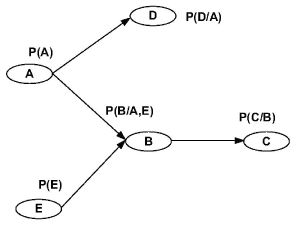.
Figure 1: A simple Bayesian network structure. View Figure 1.

In , the dynamic Bayesian network with external noise from time series is introduced. The influence of external noise on the systems dynamics is due to flipping of the value of a gene ${X}_{i}$ at each time step with a probability ${P}_{\epsilon }$. The result shows that increasing the value of external noise can reduce the overall performance.

Linear additive regulation model with noise

In this model the expression level of a gene at a certain point can be calculated by the weighted sum of the expression levels of all genes in the network at a previous time point . It may be represented by ODEs

Where ${e}_{i}$ is the gene expression level of the ${i}^{th}$ gene. N is the number of genes in the GRN. ${w}_{ij}$ represents the effect of the ${j}^{th}$ gene on the ${i}^{th}$ gene. Negative ${w}_{ij}$ means inhibition while positive ${w}_{ij}$ represents activation. ${u}_{k}$ is the ${k}^{th}$ external (control) variable. ${\nu }_{ik}$ represents the effect of the ${k}^{th}$ external variable on the ${i}^{th}$ gene. k is the number of external variable. $\beta$ is a bias term. In , the noise in the input data is considered in the linear additive regulation model. For each new input data, there is small amount of Gaussian noise with the same standard deviation added.

Neural networks model with noise

Neural networks model uses differential equations to describe GRN .

Where $f$ is usually a nonlinear function, such as a sigmoidal function. $w$ is the weight matrix. ${u}_{k}$ is the ${k}^{th}$ external (control) variable. ${\nu }_{ik}$ represents the effect of the ${k}^{th}$ external variable on the ${i}^{th}$ gene. The constant ${\lambda }_{i}$ represents the rate constant of degradation of the gene product $i$. ${\beta }_{i}$ represents an external input. Neural networks can be used to assimilate the microarray data and construct GRNs . In , Hierarchical Bayesian Neural Network model was introduced. Two kinds of noise are considered: independent parameters with Gaussian noise and correlated parameters with Multivariate Normal distribution. In , stochastic neural network models are presented for gene regulatory networks. The Poisson random noise is used to represent chance events in the process of synthesis. For expression data with normalized concentrations, exponential or normal random noise is used to generate the synthetic data.

Continuous Nonlinear Ordinary Differential Equations Model with Noise

A continuous nonlinear ordinary differential equation model is adopted. It includes random noise parameters from intrinsic noise and external noise which come from the noise source from gene regulating process. Compared to linear models, identification of the nonlinear ordinary equation model is computationally more intensive and can require more data; however, the range of nonlinear behaviors exhibited by GRNs can be more thoroughly understood with nonlinear differential equations. With more time-series data become available owing to advances in microarray or other biological technologies, and assuming continued improvement in computational capacity, it can be expected that continuous dynamic model will play a critical role in revealing complicated gene behavior.

Assuming there are N genes of interest and ${x}_{i}$ denotes the state (such as the microarray reading) of the ${i}^{th}$ gene, then the dynamics of the GRN may be modeled as

where ${\nu }_{i}$ is external noise.

In this study we assume the functions (${f}_{i},\text{\hspace{0.17em}}\forall i$ ) are in the form

where ${\Omega }_{ij}\left({x}_{1},{x}_{2},\cdots ,{x}_{N}\right)$ is the ${j}^{th}$ component of the nonlinear function ${f}_{i}$, ${L}_{i}$ is the number of components in $\Omega$ function. ${\mu }_{ij}$ is parameter noise.

The proposed model includes all the major characteristics of a gene regulatory network: it is nonlinear, dynamic, and noisy. The rationale behind the proposed model are two-fold: first, the proposed model is general and sufficiently flexible to include many well-known models and new models yet to be found; second, the noisy nature of GRNs is modeled explicitly. The deterministic model (without noise) corresponds to the nominal case, while the various stochastic effects are included as noise disturbances. Previous work has modeled these noise types by Gaussian white noise processes . The inclusion of noise also enables the proposed model to provide interpretation of the fact that GRNs are robust to noise, by which it is meant that the relationships among genes are not greatly affected by small changes caused by noise.

The nonlinear (${f}_{i},\text{\hspace{0.17em}}\forall i$) need to be identified from time-series microarray measurements such that the identification error is minimized and the simplest model structure is selected. Both synthetic data and experimental data from microarray measurements are used to evaluate the proposed method. Note that although the proposed method is tested only using polynomials as the nonlinear terms, it is expected that it should perform similarly well for other choices of nonlinear terms in the proposed model, dependent of course on sufficient data for more complex nonlinear models.

The Algorithm for Noise Analysis in Polynomial Model, S-system model, and Microarray Data

In this section, the polynomial model, S-system model, and particle filter are described in details. The algorithm for noise analysis inside gene regulatory networks is proposed on the basis of particle filter. The key steps of the proposed algorithm are shown in figure 2. The proposed algorithm is shown in details below.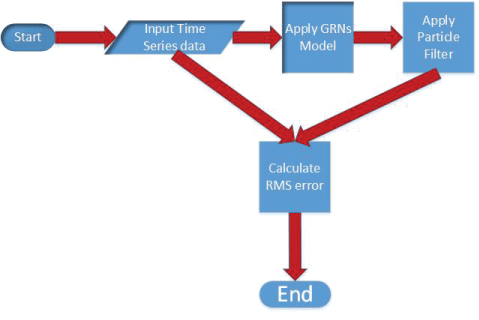.
Figure 2: Block diagram of noise analysis using particle filter. View Figure 2.

Polynomial model

In this research, polynomials are chosen as the nonlinear components (${\Omega }_{ij}\left({x}_{1},{x}_{2},\cdots ,{x}_{N}\right)$ ) in the proposed model and ODEs with dynamic polynomials are used in our test cases. The polynomials are utilized as universal approximators. In order to mitigate the effect of "the curse of dimensionality", only second-degree polynomials are selected. Note that an advantage of using low-degree polynomial models is that even when there exists some model mismatch, these models may be sufficiently accurate to represent many real systems, and thus are widely utilized in practice . We note that a similar GRN model has been adopted by , but without noise being included in the model. The polynomial model is given by:

$\begin{array}{c}\frac{d{x}_{i}}{dt}=\sum _{i=1}^{N}\text{\hspace{0.17em}}{\alpha }_{i}{x}_{i}+\sum _{i=1}^{N}\text{\hspace{0.17em}}\sum _{j=1}^{N}\text{\hspace{0.17em}}{\beta }_{i,j}{x}_{i}{x}_{j}+\sum _{i=1}^{N}\text{\hspace{0.17em}}\sum _{j=1}^{N}\text{\hspace{0.17em}}\sum _{k=1}^{N}\text{\hspace{0.17em}}{\gamma }_{i,j,k}{x}_{i}{x}_{j}{x}_{k}\\ +\cdots +{\nu }_{i}\end{array}$

where N is the number of genes, ${x}_{i}$ is the gene, ${i}^{th}$, ${\alpha }_{i}$ , ${\beta }_{i,j}$ and ${\gamma }_{i,j,k}$ are constant factors.

S-system model

Inference of GRNs using S-system model from time-series microarray measurement data has attracted a lot of attention recently [27-30]. The S-system model is given by:

$\frac{d{x}_{i}}{dt}={\alpha }_{i}\prod _{j=1}^{N}\text{\hspace{0.17em}}{\left({x}_{j}+{\mu }_{i,j}\right)}^{{g}_{i,j}}-{\beta }_{i}\prod _{j=1}^{N}\text{\hspace{0.17em}}{\left({x}_{j}+{\mu }_{i,j}\right)}^{{h}_{i,j}}+{\nu }_{i}$

where $i$ is the index for the genes $i=1,...,N$, ${x}_{i}$ are the state variables, ${\alpha }_{i}$ and ${\beta }_{i}$ are the positive rate constants, and ${g}_{i,j}$ and ${h}_{i,j}$ are the exponential parameters called kinetic orders. If ${g}_{i,j}>0$, gene $j$ will induce the expression of gene $i$. On the contrary, gene $j$ will inhibit the expression of gene $i$ if ${g}_{i,j}<0$. ${h}_{i,j}$ will have the opposite effects on controlling gene expressions compared to ${g}_{i,j}$. The S-system is a quantitative model which is characterized by power-law functions. It has a rich structure capability of capturing various dynamics in many biochemical systems. In addition, the S-system model has been proven to be successful in modeling GRNs [30,28]. Hence, the S-system model is adopted for modeling GRNs in this study. Because the microarray data usually contain noise, it is very hard to pinpoint the exact values of the parameters. Hence, the determined parameters in the system were seen as some kind of distributions .

Particle filter

Particle filter is also called Sequential Monte Carlo (SMC) methods. Particle filter is a set of genetic-type particle Monte Carlo methodologies to solve the filtering problem . It is a probability-based filter. The key idea is to generate a given number $M$ state vectors based on the probability density function (pdf) . In particle filter, the microarray data is represented in terms of the variables ${y}_{k}$, which are also a set of noisy observations. A state space model is given below.

${x}_{i}=f\left({x}_{i-1},{w}_{i-1}\right)+{\nu }_{i}$

${y}_{i}=h\left({x}_{i}\right)+{\mu }_{i}$

The covariance of ${\mu }_{i}$ is $R$ , and the covariance of ${\nu }_{i}$ is $Q$.

The general procedure of particle filter is given by Algorithm 1.

Algorithm 1: The noise process of GRNs model on the basis of particle filter

Input: S-system function $f,{\mu }_{i,j},{\nu }_{i}$, the initial stage pdf (x0)

1: Generate M initial posteriori particles on the basis of the pdf (x0): ${x}^{+}{}_{0,j}\left(j=1,....,M\right).$ M is the number of particles.

2: for $i=1\to K$ do

3: Perform the time propagation step to obtain a priori particles ${x}^{-}{}_{i,j}$

Where ${w}^{j}{}_{i-1}$ is generated on the basis of pdf of ${w}_{k-1}$.

4: Compute the relative likelihood qj of each particle ${x}^{-}{}_{i,j}$ conditioned on the measurement of yi on the basis of non linear measurement equation (Polynomial and S-system models) and the pdf of the measurement noise

${q}_{j}=\frac{1}{{\left(2\pi \right)}^{L/2}|R|}\mathrm{exp}\left(\frac{-{\left[{y}^{*}-h\left({x}^{-}{}_{i,j}\right)\right]}^{T}{R}^{-1}\left[{y}^{*}-h\left({x}^{-}{}_{i,j}\right)\right]}{2}\right)$

Where y* is a specific measurement, and L is the number of elements.

5: Scale the relative likelihoods

${q}_{j}=\frac{{q}_{j}}{\sum _{m=1}^{M}{q}_{m}}$

6: Resampling step: generate posteriori particles ${x}^{+}{}_{i,j}$ on the basis of the relative likelihoods qi

7: Compute the mean and covariance on the basis of ${x}^{+}{}_{i,j}$ which are distributed according to the
pdf $\left({x}_{i}/{y}_{i}\right)$

8: end for

9: Estimate the RMS error

$RMS={\left(\frac{1}{{N}^{2}}\sum _{i=1}^{i=N}|{X}^{*}{}_{i}-{X}_{i}|\right)}^{1}{2}}$

where ${X}^{*}{}_{i}$ is one specific measurement.

Noise analysis of microarray data using particle filter

The operations of proposed method to analyze noise in GRNs model using particle filter is graphically depicted in figure 2. The corresponding pseudocode of our method is summarized in Algorithm 2

Algorithm 2: The simulation procedure of GRNs model using particle filter

1: Generate data without any noise using Runge Kutta algorithm

Where h is the time step.

2: Generate data with system noise (Covariance is Q) and measurement noise (Covariance is R)

3: Apply particle filter on the noisy data to estimate the GRNs

4: Estimate the RMS error

$RMS={\left(\frac{1}{{N}^{2}}\sum _{i=1}^{i=N}|{X}^{*}{}_{i}-{X}_{i}|\right)}^{1}{2}}$

Synthetic Simulation Results

The noise analysis method for GRNs models is tested by synthetic data using polynomial and S-system GRNs models.

Synthetic data simulation using polynomial model

In order to test the noise analysis procedure using particle filter, the synthetic network using polynomial model can be approximated as:

${x}_{1}=-10.32{x}_{1}{x}_{3}$

${x}_{2}=9.72{x}_{1}{x}_{3}-17.5{x}_{2}$

${x}_{3}=-9.7{x}_{1}{x}_{3}+17.5{x}_{2}$

Figure 3, Figure 4, and Figure 5 show simulation results by particle filter. Genes $x1$, $x2$, and $x3$ can be inferred under external and internal noise environment which make sure to find the relations among genes.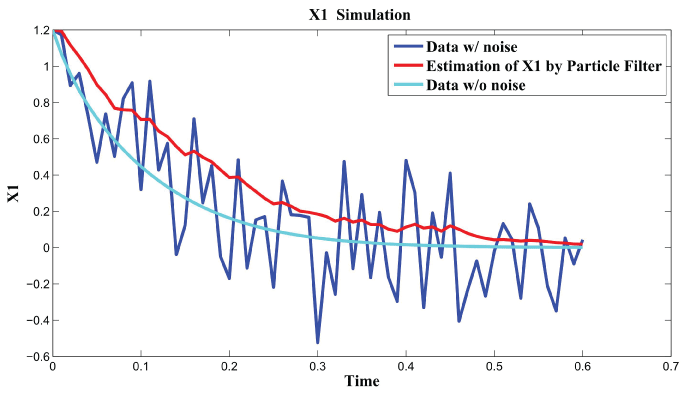.
Figure 3: Synthetic simulation data for X1 with Noise Covariance Q = 10 and . View Figure 3..
Figure 4: Synthetic simulation data for X2 with Noise Covariance Q = 10 and R = 0.08. View Figure 4.

In order to further analyze the noise relation to final results, RMS error is used to testify the effects of different noise level with $Q\in \left(0,20\right)$ and $R\in \left(0,0.1\right)$. The result is shown in figure 6. From the data and figure 6, we can see that RMS error is distributed within the interval $\left[11.34,18.10\right]$ when $R$ is in the range $\left[0,0.1\right]$. RMS error is distributed within the interval $\left[11.18,12.04\right]$ when $Q$ is in the range $\left[0,20\right]$. We concluded that the effect of noise $R$ is larger than the effect of noise $Q$ on the final results. In the research of , the observation that the time scale for intrinsic noise fluctuations is much shorter than that for extrinsic noise suggests that extrinsic noise may affect cellular phenotype more strongly than intrinsic noise at least in E. coli. Our results further confirmed this conclusion.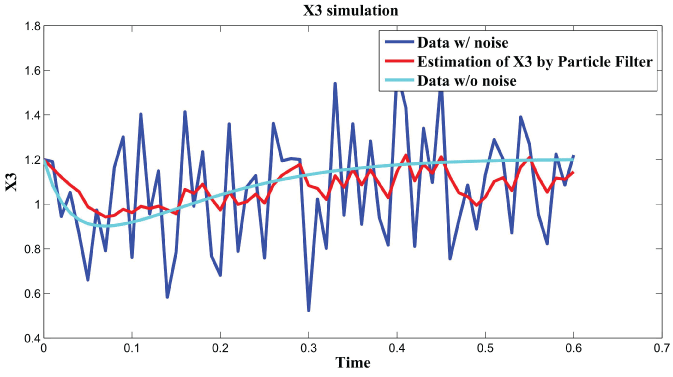.
Figure 5: Synthetic simulation data for X3 with Noise Covariance Q = 10 and R = 0.08. View Figure 5.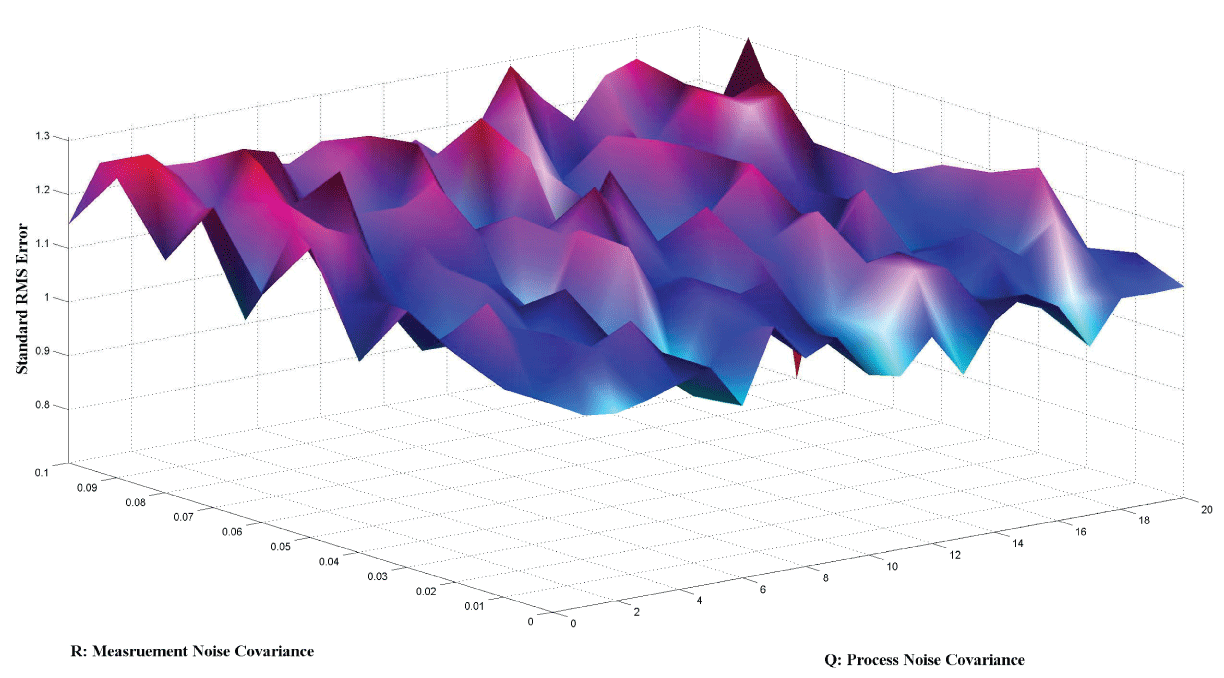.
Figure 6: The relations of Q and R to Standard RMS Error using polynomial model. View Figure 6.

Synthetic data simulation using S-system model

In order to examine the effectiveness of the proposed procedures for noise analysis in S-system using particle filter, a synthetic S-System model is used. The original S-system model is given as follows .

$\begin{array}{l}\stackrel{.}{{x}_{1}}={x}_{2}^{-2.49}-{x}_{1}^{0.12}\\ \stackrel{.}{{x}_{2}}={x}_{1}^{2.51}-{x}_{2}^{0.12}\end{array}$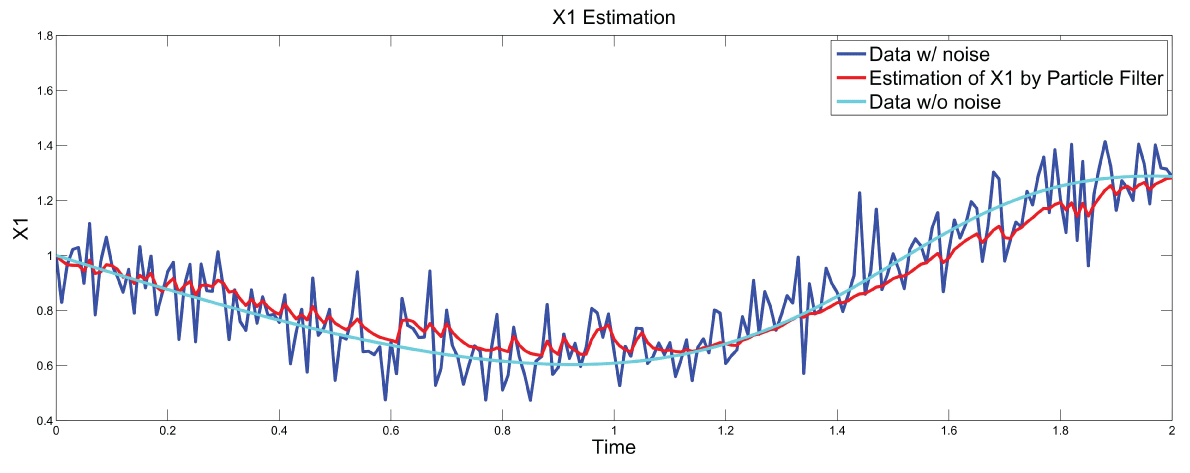.
Figure 7: Synthetic simulation data for X1 with Noise Covariance Q = 10 and R = 0.1. View Figure 7.

Figure 7 and figure 8 show simulation results using particle filter with noise levels by Q = 10 and R = 0.1. In the simulation, $K$ is equal to $200$ and the number of particles $M$ is equal to $300$. We notice that particle filter can predict the system with an error so negligible that it cannot be realized by linear filters. Figure 9 shows the relations among RMS error, process noise covariance, and measurement noise covariance. When measurement noise covariance increases, the RMS error increases more. This indicates that the increase of measurement noise covariance can incur much more error than the process noise covariance.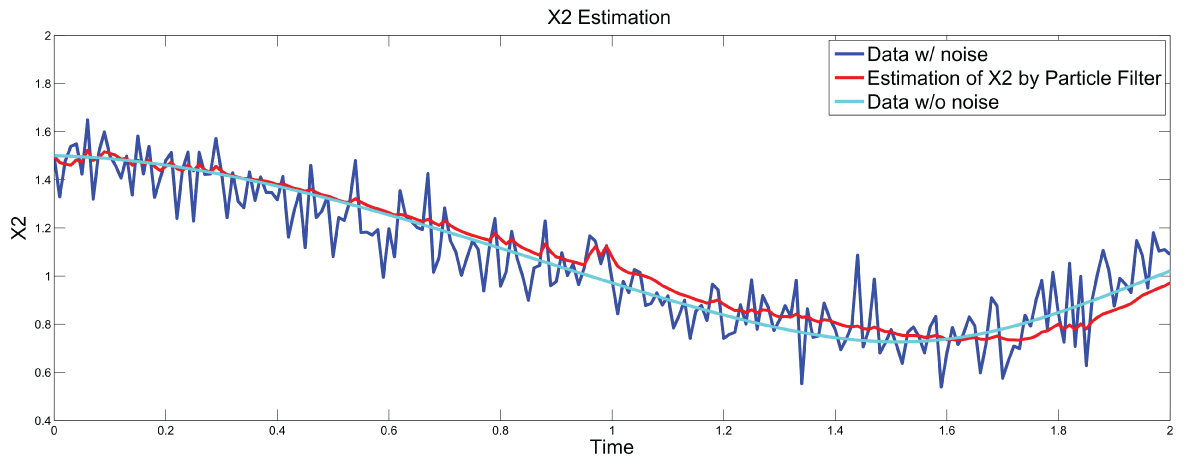.
Figure 8: Synthetic simulation data for X2 with Noise Covariance Q = 10 and R = 0.1 View Figure 8.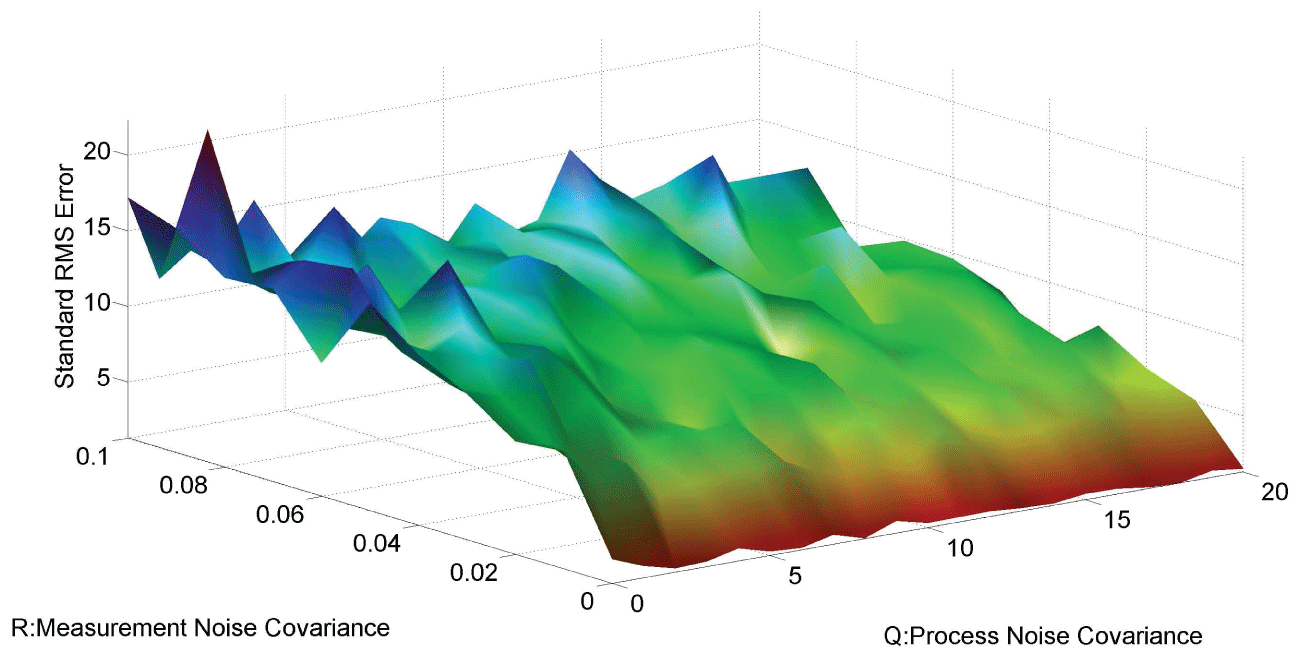.
Figure 9: The relations of Q and R to Standard RMS Error using S-system model. View Figure 9.

Microarray Data Simulation

During this part of the simulation, time-series gene-expression data corresponding to yeast protein synthesis  are considered. Five genes (HAP1 (${x}_{1}$), CYB2 (${x}_{2}$), CYC7 (${x}_{3}$), CYT1 (${x}_{4}$), COX5A (${x}_{5}$)) are selected because the relations among them have been revealed by biological experiments. The relations are shown in figure 10 with noise.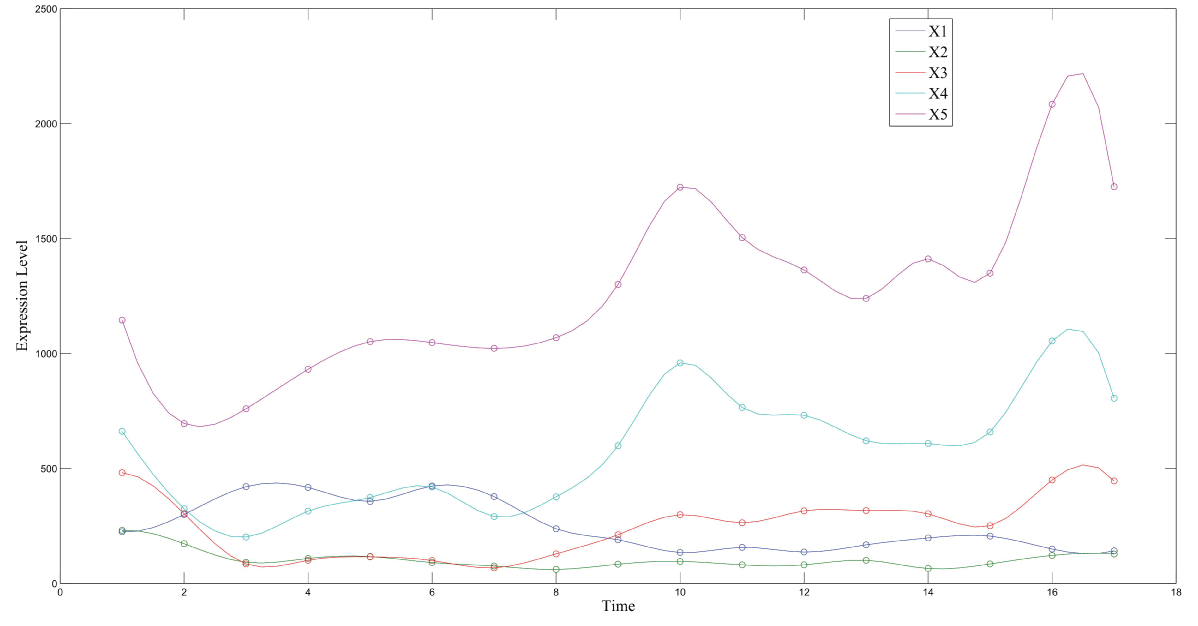.
Figure 10: The microarry data with noise in S-system model. View Figure 10.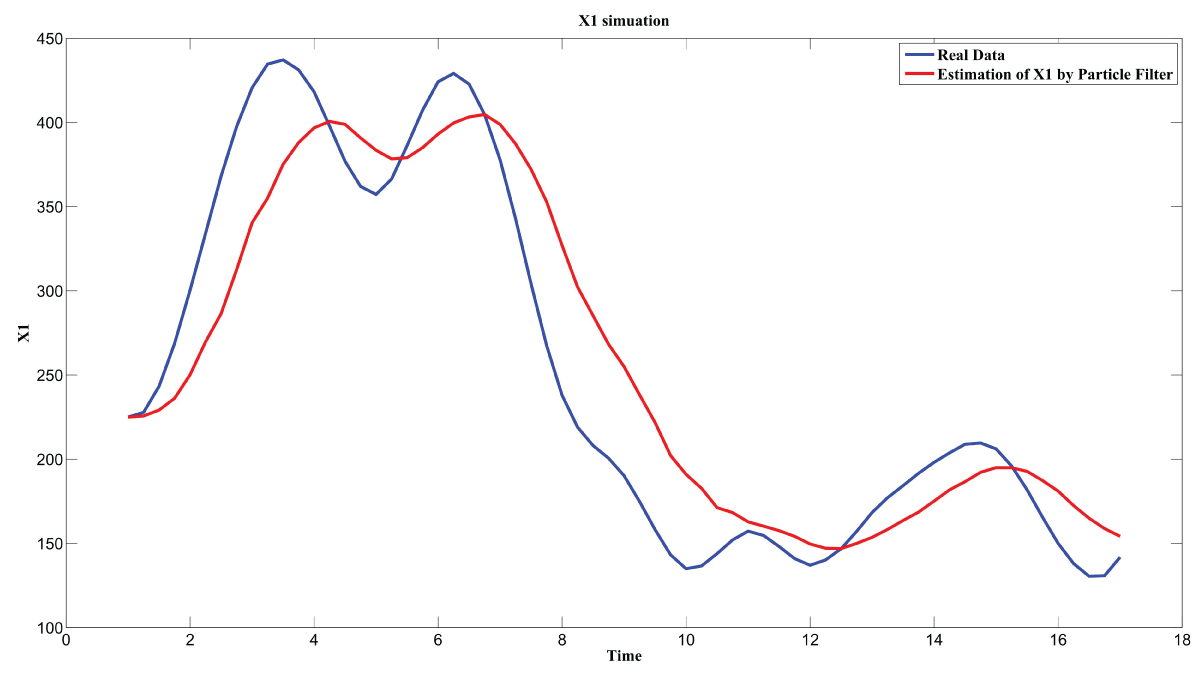.
Figure 11: Microarray data simulation for HAP1. View Figure 11.

Particle filter is applied to analyze the data with noise. The particle filter estimate is the mean of particles which caused the time shift or delay on the curve. It is inherent in particle filter and is called mean shift.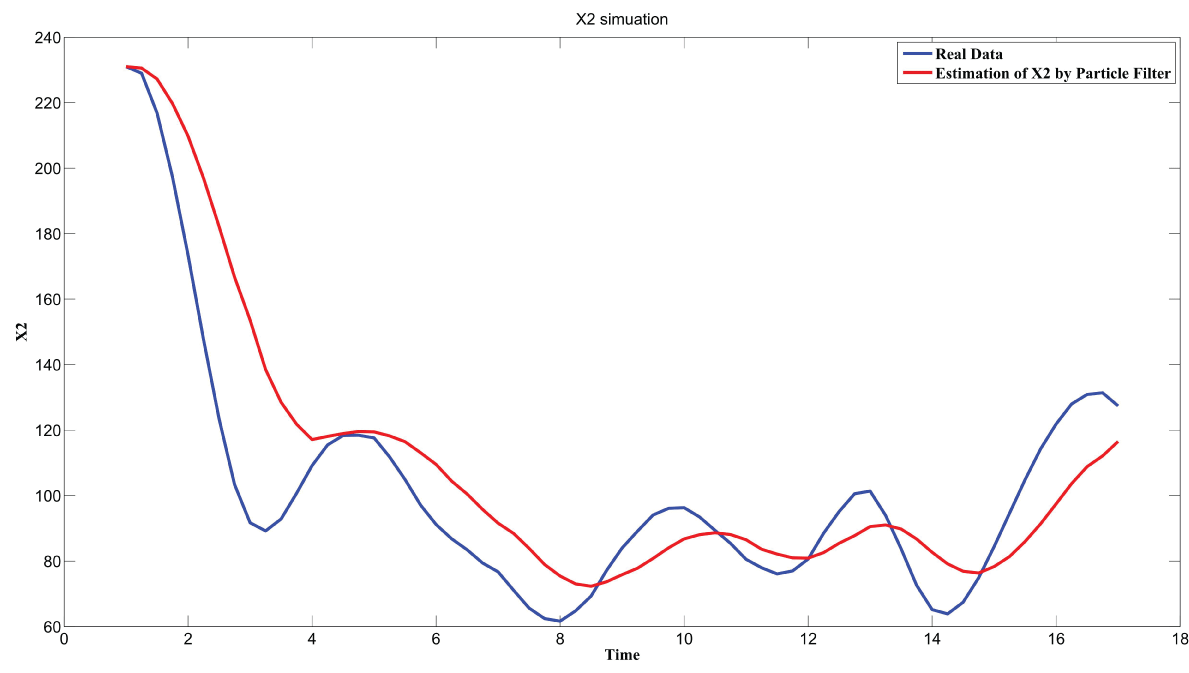.
Figure 12: Microarray data simulation for CYB2. View Figure 12.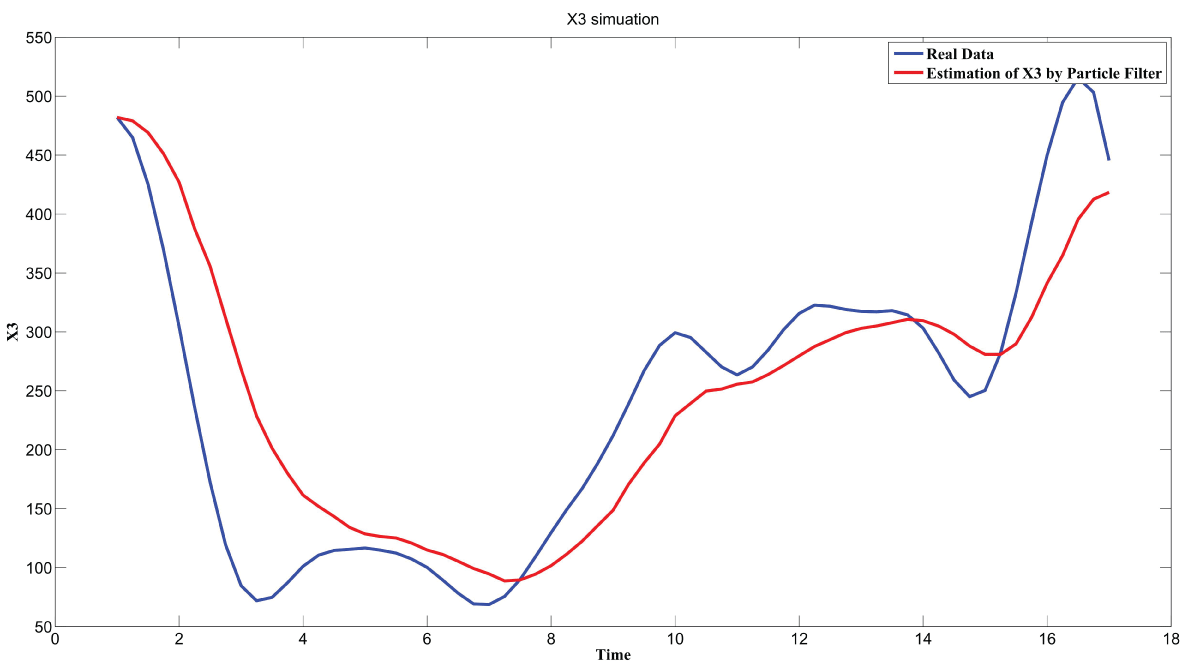.
Figure 13: Microarray data simulation for CYC7. View Figure 13.

The result is shown by figure 11, figure 12, figure 13, figure 14, and figure 15 for genes HAP1, CYB2, CYC7, CYT1 and COX5A. The branch pathway model is shown by figure 16 on the basis of the above results.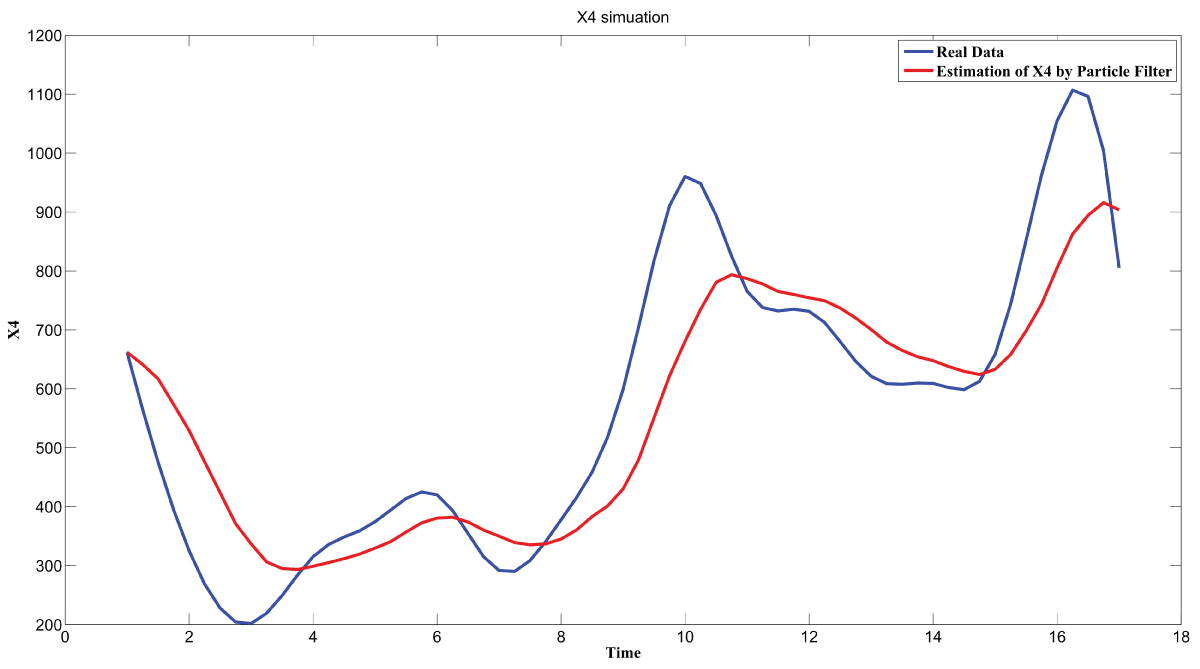.
Figure 14: Microarray data simulation for CYT1. View Figure 14.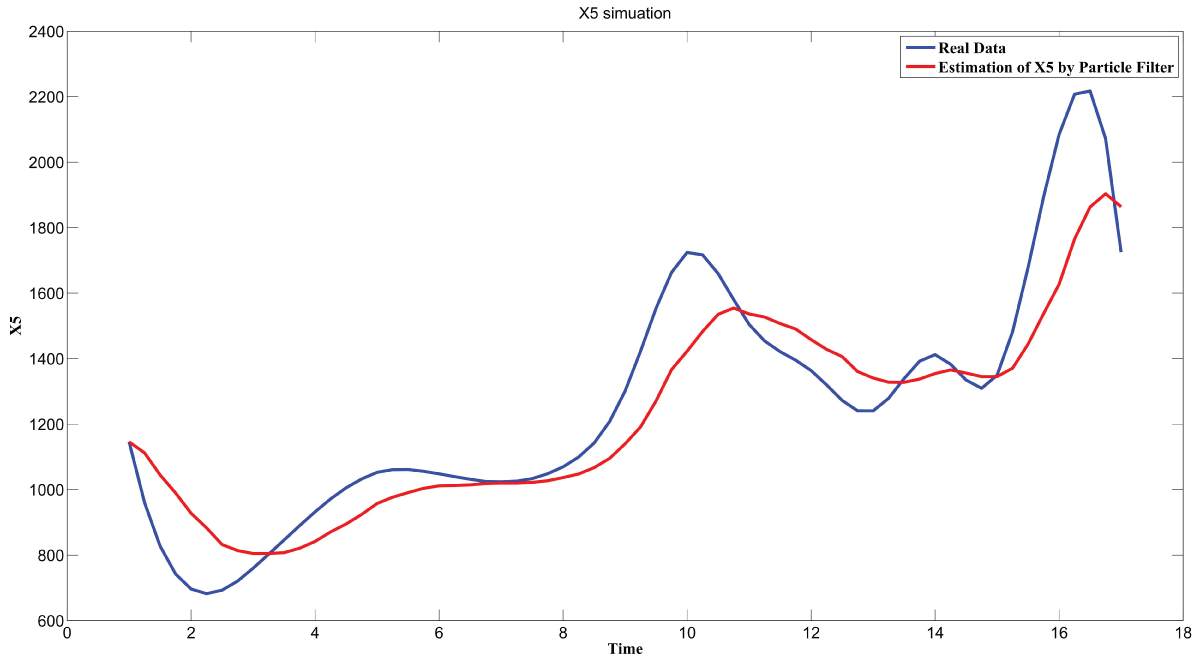.
Figure 15: Microarray data simulation for COX5A. View Figure 15.

The relations are in agreement with the biological experiment findings in .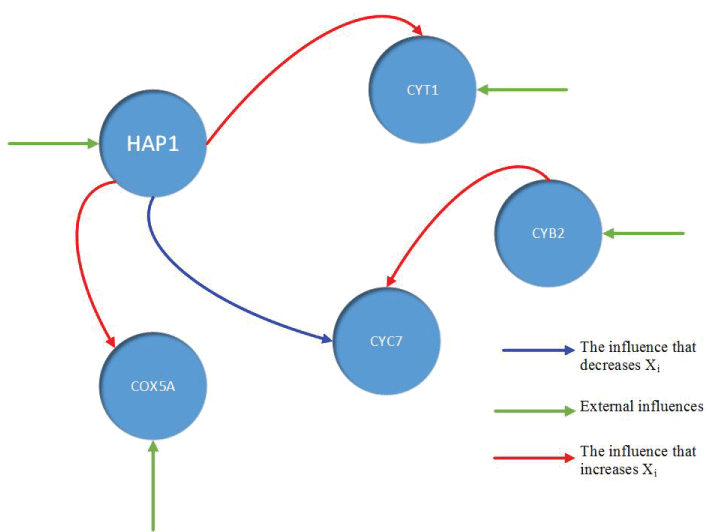.
Figure 16: The branch pathway model for genes HAP1, CYB2, CYC7, CYT1, and COX5A. View Figure 16.

Conclusions

In this paper, process noise and measurement noise of gene regulatory networks is analyzed using particle filter. The synthetic models on the basis of polynomial and S-system are studied to analyze the relations among RMS, measurement noise, and process noise. We found out that measurement noise is the main reason to incur the RMS error conforming to results from biological experimental research. Noise inside microarray data is considered and analyzed with five real genes.

Acknowledgement

The work of Haixin Wang and Dawit Aberra was supported by NSF Grant 1435152.

ReferencesOpen Access by ClinMed International Library is licensed under a Creative Commons Attribution 4.0 International License based on a work at https://clinmedjournals.org/.